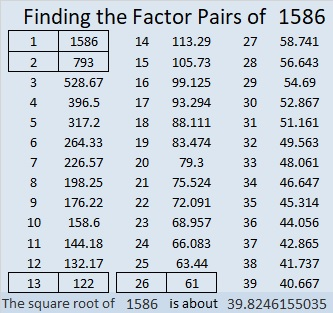# 1586 and a Level One Puzzle

Contents

### Today’s Puzzle:

Write the number from 1 to 12 in both the first column and the top row of the puzzle so that the given clues are the products of the numbers you write.### Factors of 1586:

• 1586 is a composite number.
• Prime factorization: 1586 = 2 × 13 × 61.
• 1586 has no exponents greater than 1 in its prime factorization, so √1586 cannot be simplified.
• The exponents in the prime factorization are 1, 1, and 1. Adding one to each exponent and multiplying we get (1 + 1)(1 + 1)(1 + 1) = 2 × 2 × 2 = 8. Therefore 1586 has exactly 8 factors.
• The factors of 1586 are outlined with their factor pair partners in the graphic below.### More about the Number 1586:

1586 is the sum of two squares in two different ways:
35² + 19² = 1586, and
31² + 25² = 1586.

1586 is the hypotenuse of FOUR Pythagorean triples:
286-1560-1586, which is 26 times (11-60-61),
336-1550-1586, calculated from 31² – 25², 2(31)(25), 31² + 25²,
610-1464-1586, which is (5-12-13) times 122, and
864-1330-1586, calculated from 31² – 25², 2(31)(25), 31² + 25².

This site uses Akismet to reduce spam. Learn how your comment data is processed.